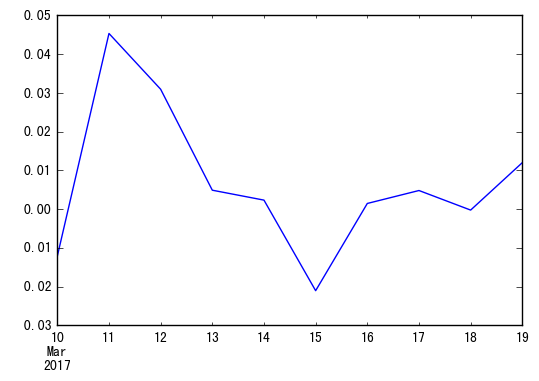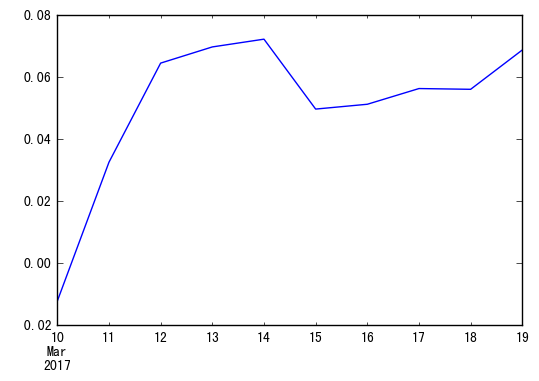# 风险指标的计算 (empyrical模块)

## 安装

``````pip install empyrical
``````

## 使用

### 导入

``````from empyrical import (
alpha,
beta,
alpha_beta_aligned,
annual_volatility,
cum_returns,
annual_return,
downside_risk,
information_ratio,
max_drawdown,
sharpe_ratio,
sortino_ratio,
calmar_ratio,
omega_ratio,
tail_ratio
)
``````

### 初始数据

#### 策略收益率

``````...
2015-07-16    -0.012143
2015-07-17    0.045350
2015-07-20    0.030957
2015-07-21    0.004902
...
``````

``````import pandas as pd
returns = pd.Series(
index=pd.date_range('2017-03-10', '2017-03-19'),
data=(-0.012143, 0.045350, 0.030957, 0.004902, 0.002341, -0.02103, 0.00148, 0.004820, -0.00023, 0.01201)
)
``````
``````returns
``````
``````2017-03-10   -0.012143
2017-03-11    0.045350
2017-03-12    0.030957
2017-03-13    0.004902
2017-03-14    0.002341
2017-03-15   -0.021030
2017-03-16    0.001480
2017-03-17    0.004820
2017-03-18   -0.000230
2017-03-19    0.012010
Freq: D, dtype: float64
``````
``````returns.plot()
``````
``````<matplotlib.axes._subplots.AxesSubplot at 0x7f3bd6fecda0>
``````#### 基准收益率

``````benchmark_returns = pd.Series(
index=pd.date_range('2017-03-10', '2017-03-19'),
data=(-0.031940, 0.025350, -0.020957, -0.000902, 0.007341, -0.01103, 0.00248, 0.008820, -0.00123, 0.01091)
)
``````

### 计算累计收益

``````creturns = cum_returns(returns)
``````
``````creturns
``````
``````2017-03-10   -0.012143
2017-03-11    0.032656
2017-03-12    0.064624
2017-03-13    0.069843
2017-03-14    0.072348
2017-03-15    0.049796
2017-03-16    0.051350
2017-03-17    0.056417
2017-03-18    0.056174
2017-03-19    0.068859
Freq: D, dtype: float64
``````
``````creturns.plot()
``````
``````<matplotlib.axes._subplots.AxesSubplot at 0x7f3bd65eb0b8>
``````### 计算最大回撤

``````max_drawdown(returns)
``````
``````-0.02103000000000009
``````

### 计算年化收益

``````annual_return(returns)
``````
``````4.3554273608590925
``````

### 年化Volatility (策略波动率)

``````annual_volatility(returns, period='daily')
``````
``````0.3055933840036463
``````

### Calmar比率

Calmar比率描述的是收益和最大回撤之间的关系。计算方式为年化收益率与历史最大回撤之间的比率。Calmar比率数值越大，基金的业绩表现越好。反之，基金的业绩表现越差。

``````calmar_ratio(returns)
``````
``````207.10543798664165
``````

### Omega比率

``````omega_ratio(returns=returns, risk_free=0.0001)
``````
``````3.0015132184078577
``````

### Sharpe比率

``````sharpe_ratio(returns=returns)
``````
``````5.6451366106126715
``````

### sortino比率

``````sortino_ratio(returns=returns)
``````
``````14.150708210667487
``````

### 下降风险

``````downside_risk(returns=returns)
``````
``````0.12191025172150209
``````

### 信息比率(Information Ratio)

Information Ratio = α∕ω (α为组合的超额收益，ω为主动风险)

``````information_ratio(returns=returns, factor_returns=benchmark_returns)
``````
``````0.43383172638699696
``````

### Alpha

``````alpha(returns=returns, factor_returns=benchmark_returns, risk_free=0.01)
``````
``````0.7781943501778946
``````

### Beta

``````beta(returns=returns, factor_returns=benchmark_returns, risk_free=0.01)
``````
``````0.56157656832313008
``````

### Tail Ratio

``````tail_ratio(returns=returns)
``````
``````2.2825137911495892
``````

## 其他说明

``````{'monthly':12
'weekly': 52
'daily': 252}
``````